# Machine Learning Tutorial #2: Training

## Topics: Performance Metrics, Cross Validation, Model Selection, Hyperparameter Optimization, Project Reflection, Tools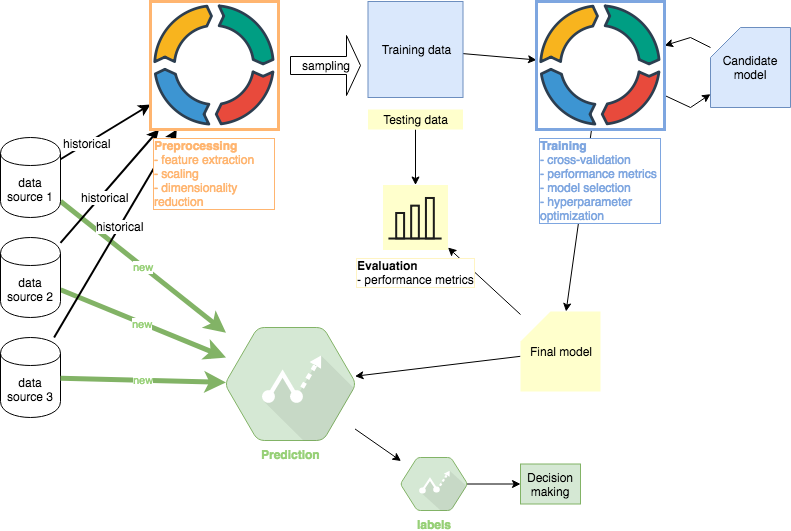Machine Learning project overview. Author: Adam Novotny

This second part of the ML Tutorial follows up on the first Preprocessing part. All code is available in this Github repo. Other tutorials in this series: #1 Preprocessing, #2 Training (this article), #3 Evaluation , #4 Prediction

I concluded Tutorial #1 with 4 datasets: training features, testing features, training target variables, and testing target variables. Only training features and and training target variables will be used in this Tutorial #2. The testing data will be used for evaluation purposes in Tutorial #3.

# Performance Metrics

In practice, a domain-specific decision could be made to supplement the standard metrics above. For example, investors are typically more concerned about significant downside errors rather than upside errors. As a result, a metric could be derived that overemphasizes downside errors corresponding to financial losses.

# Cross Validation

Our cross validation must respect the temporal sequence of the data. We can use Walk Forward Validation or simply multiple Train-Test Splits. For illustration, I will use 3 Train-Test splits. For example, let’s assume we have 2000 samples sorted by timestamp from the earliest. Our 3 segments would look as follows: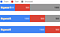Train-Test splits. Author: Adam Novotny

# Model Selection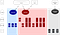ML Model Selection. Author: Adam Novotny

In this section, I will select the models to train. The “Supervised” algorithms section (red section in the image above) is relevant because the dataset contains both features and labels (target variables). I like to follow Occam’s razor when it comes to algorithms selection. In other words, start with the algorithm that exhibits the fastest times to train and the greatest interpretability. Then we can increase complexity.

I will explore the following algorithms in this section:

• Linear Regression: fast to learn, easy to interpret
• Decision Trees: fast to learn (requires pruning), easy to interpret
• Neural Networks: slow to learn, hard to interpret

## Linear Regression

Training a linear regression model using sklearn is simple:

`from sklearn import linear_modelmodel = linear_model.LinearRegression()model.fit(X_train, y_train)y_pred = model.predict(X_test)`

Initial results yielded nothing remotely promising so I took another step and transformed features further. I created polynomial and nonlinear features to account for nonlinear relationships. For example, features [a, b] become [1, a, b, a², ab, b²] in the case of degree-2 polynomial.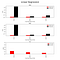Linear Regression results. Author: Adam Novotny

The x-axis represents 3 cross validation segments (the fold 1st uses 1749 samples for training and 1749 for testing, the 2nd uses 3499 for training and 1749 for testing, and the last uses 5249 for training and 1749 for testing). Clearly, the results suggest that the linear model is not useful in practice. At this stage I have at least the following options:

• Ridge regression: addresses overfitting (if any)
• Lasso linear: reduces model complexity

At this point, I don’t believe that any of the options above will meaningfully impact the outcome. I will move on to other algorithms to see how they compare.

Before moving on, however, I need to set expectations. There is a saying in finance that successful forecasters only need to be correct 51% of the time. Financial leverage can be used to magnify results so being just a little correct produces impactful outcomes. This sets expectations because we will never find algorithms that are constantly 60% correct or better in this domain. As a result, we expect low R² values. This needs to be said because many sample projects in machine learning are designed to look good, which we can never match in real-life price forecasting.

## Decision Tree

`from sklearn import treemodel = tree.DecisionTreeRegressor()model.fit(X_train, y_train)y_pred = model.predict(X_test)`

The default results for the fit function above almost always overfit. Decision trees have a very expressive hypothesis space so they can represent almost any function when not pruned. R² for training data can easily become perfect 1.0 while for testing data the result will be 0. We therefore need to use the max_depth argument of scikit-learn DecisionTreeRegressor to enforce that the tree generalizes well for test data.

One of the biggest advantages of decision trees is their interpretability: see many useful visualization articles using standard illustrative datasets.

## Neural Networks

`from sklearn.neural_network import MLPRegressormodel = MLPRegressor(hidden_layer_sizes=(200, 200), solver="lbfgs", activation="relu")model.fit(X_train, y_train)y_pred = model.predict(X_test)`

Training a neural net with 2 hidden layers (of 200 units each) and polynomial features starts taking tens of seconds on an average laptop. To speed up the training process in the next section, I will step away from scikit-learn and use Keras with TensorFlow backend.

Keras API is equally simple. The project even includes wrappers for scikit-learn to take advantage of scikit’s research libraries.

`from keras.models import Sequentialfrom keras.layers import Densemodel = Sequential()input_size = len(X)model.add(Dense(200, activation="relu", input_dim=input_size))model.add(Dense(200, activation="relu"))model.add(Dense(1, activation="linear"))model.compile(optimizer="adam", loss="mse")model.fit(X_train, y_train, epochs=25, verbose=1)y_pred = model.predict(X_test)`

# Hyperparameter Optimization

## Decision Tree

• splitter: strategy used to split nodes (best or random)
• max depth of the tree
• min samples per split: the minimum number of samples required to split an internal node
• max leaf nodes: number or None (allow unlimited number of leaf nodes)

Illustrative grid search results are below: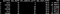Grid Search Decision Tree — first rows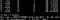Grid Search Decision Tree — last rows

Performance using the best parameters: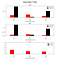Decision Tree results

Again, the results do not seem to be very promising. They appear to be better than linear regression (lower MAE and MSE) but R² is still too low to be useful. I would conclude, however, that the greater expressiveness of decision trees is useful and I would discard the linear regression model at this stage.

## Neural Networks

• number of hidden layers and/or units in each layer
• model optimizer (SGD, Adam, etc)
• activation function in each layer (relu, tanh)
• batch size: the number of samples per gradient update
• epochs to train: the number of iterations over the entire training dataset

Illustrative grid search results are below: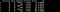Grid Search Neural Net — first rows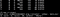Grid Search Neural Net — last rows

Using the best parameters, we obtain the following performance metrics: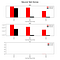Keras MAE, MSE, R2

Neural net and decision tree results are similar which is common. Both algorithms have very expressive hypothesis spaces and often produce comparable results. If I achieve comparable results, I tend to use the decision tree model for its faster training times and greater interpretability.

# Project Reflection

As I mentioned in the first Preprocessing Tutorial, finance practitioners might spend months sourcing data and building features. Domain-specific knowledge is crucial and I would argue that financial markets exhibit at least the Weak-Form of Efficient Market Hypothesis. This implies that future stock returns cannot be predicted from past price movements. I have used only past price movements to develop the models above so practitioners would notice already in the first tutorial that results would not be promising.

For the sake of completing this tutorial, I will go ahead and save the decision tree model and use it for illustrative purposes in the next sections of this tutorial (as if it were the Final production model):

`pickle.dump(model, open("dtree_model.pkl", "wb"))`

Important: there are known security vulnerabilities in the Python pickle library. To stay on the safe side, the key takeaway is to never unpickle data you did not create.

# Tools

Other tutorials in this series: #1 Preprocessing, #2 Training (this article), #3 Evaluation , #4 Prediction

Author website: adamnovotny.com

## Coinmonks

Coinmonks is a non-profit Crypto educational publication.

## Coinmonks

Coinmonks is a non-profit Crypto educational publication. Follow us on Twitter @coinmonks Our other project — https://coincodecap.com## Coinmonks

Coinmonks is a non-profit Crypto educational publication. Follow us on Twitter @coinmonks Our other project — https://coincodecap.com

## More From Medium

Medium is an open platform where 170 million readers come to find insightful and dynamic thinking. Here, expert and undiscovered voices alike dive into the heart of any topic and bring new ideas to the surface. Learn more

Follow the writers, publications, and topics that matter to you, and you’ll see them on your homepage and in your inbox. Explore

If you have a story to tell, knowledge to share, or a perspective to offer — welcome home. It’s easy and free to post your thinking on any topic. Write on Medium